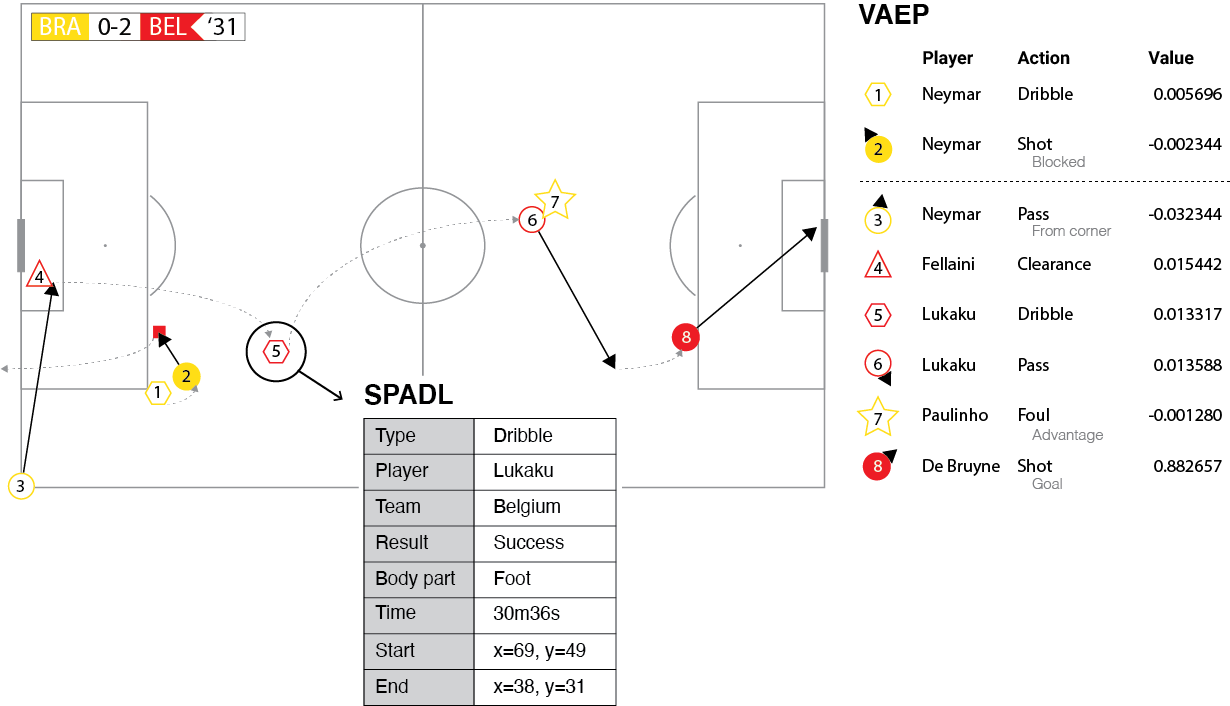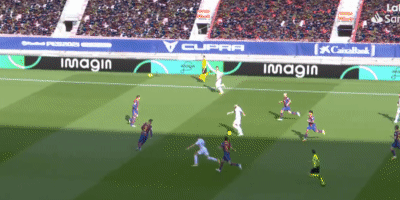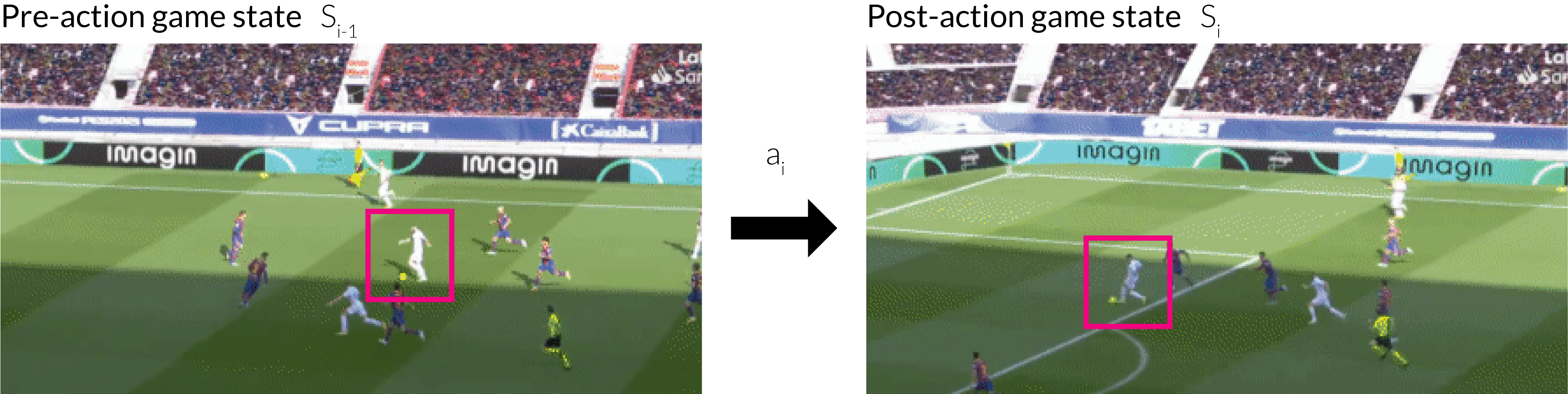# Valuing actions#

Once you’ve collected the data and converted it to the SPADL format, you can start valuing the contributions of soccer players. This document gives a general introduction to action valuing framweworks and links to a detailled discussion of the three implmented frameworks.

## General idea#

When considering event stream data, a soccer match can be viewed as a sequence of $$n$$ consecutive on-the-ball actions $$\left[a_1, a_2, \ldots, a_n\right]$$ (e.g., [pass, dribble,…, interception]). Action-valuing frameworks aim to assign a numeric value to each of these individual actions that quantifies how much the action contributed towards winning the game. This value should reflect both the circumstances under which it was performed as well as its longer-term effects. This is illustrated in the figure below:However, rather than directly assigning values to actions, the existing approaches all start by assigning values to game states. To illustrate the underlying intuition, consider the pass below:The effect of the pass was to change the game state:The figure on the left shows the game in state $$S_{i−1} = \{a_1,\dots,a_{i−1}\}$$, right before Benzema passes to Valverde and the one on the right shows the game in state $$S_i = \{a_1, \ldots, a_{i−1}, a_i\}$$ just after Valverde successfully controlled the pass.

Consequently, a natural way to assess the usefulness of an action is to assign a value to each game state. Then an action’s usefulness is simply the difference between the post-action game state $$S_i$$ and pre-action game state $$S_{i-1}$$. This can be expressed as:

$U(a_i) = V(S_i) - V(S_{i-1}),$

where $$V$$ captures the value of a particular game state.

The differences between different action-valuing frameworks arise in terms of (1) how they represent a game state $$S_i$$, that is, define features such as the ball’s location or score difference that capture relevant aspects of the game at a specific point in time; and (2) assign a value $$V$$ to a specific game state.

## Implemented Frameworks#

The socceraction package implements three frameworks to assess the impact of the individual actions performed by soccer players: xT, VAEP and Atomic-VAEP.